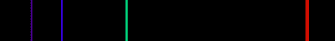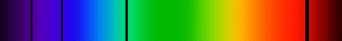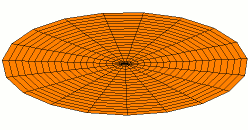# Electron moving

hi,

ive got a really simple question.
niels bohr proposed a model of the atom in witch the electron cannot fall into any energy state but must fall be in certain discreet values and this helps explain why the electron doesnt clumb with the nucleus almost instantly.

not the atom needed to have a structure with an electron separated from a massive nucleus from experimental reasons.

but if you dare to assume that an electron must have discreet energy values, why not assume a much simpler thing in the first place, namely that the electron doesn't orbit the nucleus but sits there stationary on some distance from the nucleus and so doesnt accelerate and emit radiation and thus doesnt loose energy and fall to the nucleus.

it seems to me that quantum mechanics is just a bunch of intuitive laws based on assumptions of a structure of the atom witch no one has even ever seen.

mathman
The following is from the Wkikpedia article:
http://en.wikipedia.org/wiki/Atomic_theory

Quantum physical models of the atom

Main article: Atomic orbital

In 1924, Louis de Broglie proposed that all moving particles — particularly subatomic particles such as electrons — exhibit a degree of wave-like behavior. Erwin Schrödinger, fascinated by this idea, explored whether or not the movement of an electron in an atom could be better explained as a wave rather than as a particle. Schrödinger's equation, published in 1926, describes an electron as a wavefunction instead of as a point particle. This approach elegantly predicted many of the spectral phenomena that Bohr's model failed to explain. Although this concept was mathematically convenient, it was difficult to visualize, and faced opposition. One of its critics, Max Born, proposed instead that Schrödinger's wavefunction described not the electron but rather all its possible states, and thus could be used to calculate the probability of finding an electron at any given location around the nucleus. This reconciled the two opposing theories of particle versus wave electrons and the idea of wave-particle duality was introduced. This theory stated that the electron may exhibit the properties of both a wave and a particle. For example, it can be refracted like a wave, and has mass like a particle.

A consequence of describing electrons as waveforms is that it is mathematically impossible to simultaneously derive the position and momentum of an electron. This became known as the Heisenberg uncertainty principle after the theoretical physicist Werner Heisenberg, who first described it and published it in 1927. This invalidated Bohr's model, with its neat, clearly defined circular orbits. The modern model of the atom describes the positions of electrons in an atom in terms of probabilities. An electron can potentially be found at any distance from the nucleus, but, depending on its energy level, exists more frequently in certain regions around the nucleus than others; this pattern is referred to as its atomic orbital. The orbitals come in a variety of shapes-sphere, dumbbell, torus, etc.-with the nucleus in the middle.

ZapperZ
Staff Emeritus
it seems to me that quantum mechanics is just a bunch of intuitive laws based on assumptions of a structure of the atom witch no one has even ever seen.

And how much quantum mechanics have you actually studied to be able to make that kind of a judgement?

QM did NOT start from such an assumption. Look at how we set up to solve for the Hydrogen atom! We set up the Hamiltonian/Schrodinger equation and set up the appropriate boundary conditions. That's it! We never assumed any kind of quantization or discrete levels. It came out of the solutions naturally!

If you have done QM and have seen this derived, then your question is puzzling. If you haven't done such a derivation, then your conclusion is faulty since it is not based on actual knowledge of QM.

Zz.

ok , thank you for copy paste, but this dont answer my question.

the reason why electrons are assumed to be separated from the nucleus ia because of a bombardment experiment with positive particles and because most of them passed freely through the material.
the conclusion was that there must be a massive positively charged core and mostly empty space and then some negatively charged particle to account for a neutral atom.

no one said that the electron has to orbit and loose energy.

so is there any explanation for why everybody assumed that electron orbits?

And how much quantum mechanics have you actually studied to be able to make that kind of a judgement?

QM did NOT start from such an assumption. Look at how we set up to solve for the Hydrogen atom! We set up the Hamiltonian/Schrodinger equation and set up the appropriate boundary conditions. That's it! We never assumed any kind of quantization or discrete levels. It came out of the solutions naturally!

If you have done QM and have seen this derived, then your question is puzzling. If you haven't done such a derivation, then your conclusion is faulty since it is not based on actual knowledge of QM.

Zz.

i dont mind about discreet values really what i mind is that the electron has to move around and loose energy, why is this necessary?

HallsofIvy
Homework Helper
so is there any explanation for why everybody assumed that electron orbits?
Well, there's your fundamental problem (and probably why zapperZ asked how much quantum physics you had studied). To the contrary, no one (at least not quantum physicists) believes that electrons actually orbit the nucleus. There is experimental evidence that an atom has a small, positively charged, nucleus but the electrons do NOT orbit, they are in "clouds" around the nucleus. The "planetary model" with electrons in orbit around a positively charged nucleus, like planets around the sun, was replaced in, I think, the 1930s.

Gold Member
but if you dare to assume that an electron must have discreet energy values, why not assume a much simpler thing in the first place, namely that the electron doesn't orbit the nucleus but sits there stationary on some distance from the nucleus and so doesnt accelerate and emit radiation and thus doesnt loose energy and fall to the nucleus.

it seems to me that quantum mechanics is just a bunch of intuitive laws based on assumptions of a structure of the atom witch no one has even ever seen.

Well... unless you can provide a peer reviewed paper, rigorously refuting Heisenberg's[/PLAIN] [Broken] uncertainty principle (your electron has simultaneously known momentum & position), and rigorously explain the existence of hyperfine structure in spectral lines due to the Spin–orbit interaction, and rigorously explain the Zeeman effect, and finally a complete explanation of doublets, triplets and multi-electron atoms spectral lines.... it seems to me that your assumption is just a bunch of ignorant claptrap, based on personal speculations.

Last edited by a moderator:
ZapperZ
Staff Emeritus
i dont mind about discreet values really what i mind is that the electron has to move around and loose energy, why is this necessary?

It doesn't move around and lose energy!

Your starting point, which is your understanding of what QM description of atoms, IS FAULTY. You are asking us to explain your faulty knowledge of QM. I don't have the patience to do that the SAME way you do not have the patience to actually learn QM before making such conclusion.

Look at the Schrodinger Equation for the Hydrogen atom. Point out to me where there is an "orbit". Until you can do that, this is doing nowhere.

Zz.

WannabeNewton
i dont mind about discreet values really what i mind is that the electron has to move around and loose energy, why is this necessary?

That's a consequence of the classical Maxwell equations and more specifically the non-relativistic Larmor formula-one can, with a bit of work, calculate the lifespan of an electron in circular orbit about the nucleus before it spirals into the nucleus due to Larmor radiation. See for example problem 11.14 in Griffiths "Introduction to Electrodynamics". However this lifespan is, as noted, derived from the classical Maxwell equations-in other words the radiation inspiral is a classical effect. Obviously this doesn't happen in the real world. As Zz already noted multiple times if you study the bound states of the electron in the hydrogen atom you will see precisely how the classical picture gets replaced. The key point is these bound state solutions to Schrodinger's equation give rise to discrete allowed energy levels.

alright, but im talking pre Schrodinger and pre Heisenberg principle.
when people did assume that classical electrodynamics must explain the atom structure.

when the atom was planetary, it was assumed that electrons orbit the nucleus at discrete values by niels bohr in order to explain the radiation of purely heated bodies or black body radiation. so electrons can only make discrete jumps and so give of discrete electromagnetic chunks of radiation, and that the electron needs a push in order to change orbit and emit.

this helped explain both the observed radiation of black bodies and the stability of atoms.

but in order to have a problem of stability of the atom one has to assume that electrons orbit.

i dont think that orbiting electrons are necessary for explaining black bodies, but maybe im wrong.

but is there any phenomenon that requires an orbiting electron at al cost?

thats my question in the first place it seems that there is no important reason that electrons orbit, but i must be wrong.

ZapperZ
Staff Emeritus
alright, but im talking pre Schrodinger and pre Heisenberg principle.
when people did assume that classical electrodynamics must explain the atom structure.

But this is ridiculous! Are you also going to question Thermodynamics because we started off with a faulty Caloric picture?

Quantum mechanics no longer hold the Bohr model. You are using something that is a preliminary model during the development of QM as if it is a true model. How do you expect us to defend such a faulty picture?

Study QM as it is NOW!

Zz.

Nugatory
Mentor
alright, but im talking pre Schrodinger and pre Heisenberg principle.
when people did assume that classical electrodynamics must explain the atom structure.
.....
but is there any phenomenon that requires an orbiting electron at all cost?

No. Never was, not "at all costs".

However, something was needed to explain why atoms have the sizes that they do. It was clear from the work of Rutherford and others that the positive charge of an atom was concentrated at the center of the atom. The electromagnetic forces between the positive-charged nucleus and the negative-charged electron are attractive, so classical electrodynamics predicted that the electrons should move in towards the nucleus unless something else acted to hold them at a distance from the nucleus. Furthermore, there was abundant evidence that free electrons behave like particles. They have distinct positions and velocities and follow classical trajectories for a point mass obeying ##F=ma##.

Thus, it seemed natural to try to explain the behavior of bound electrons in terms of positions, velocities, and trajectories; there was no obvious reason to expect that everything would be different just because the electron happens to be very close to a strong positive charge. However, this proved impossible, for all the well-known reasons: no electromagnetic radiation from a stable electron, blackbody radiation, chemical bonds and the energy associated with them, and so forth.

So to answer your question "why did people assume the atom orbited."... It was a reasonable hypothesis that was dropped when it failed to match experimental results and a hypothesis that better matched experimental data, namely quantum mechanics, came along.

However, you asked a different question in your first post: why not assume that the bound electron sits at a fixed position from the nucleus, not moving? Nowadays, we don't buy that model because quantum mechanics as we understand it today works so much better. But why didn't Bohr adopt that model back then? He's not around to ask, but I'd expect that he rejected it for the same reason his contemporaries would have: conservation of energy. A free electron in the neighborhood of a nucleus has a total energy which is the sum of its kinetic and potential energy. When it is captured by the nucleus, radiation is emitted, but the energy carried by that radiation is less than the difference between the initial energy and the final potential energy. Where could the rest of the initial energy have gone, if not into residual kinetic energy of the bound electron?

Gold Member
alright, but im talking pre Schrodinger and pre Heisenberg principle.
when people did assume that classical electrodynamics must explain the atom structure.
[...]
i dont think that orbiting electrons are necessary for explaining black bodies, but maybe im wrong.

but is there any phenomenon that requires an orbiting electron at al cost?

thats my question in the first place it seems that there is no important reason that electrons orbit, but i must be wrong.

Yes, you are terrible wrong. What you are trying to do is like; while not understanding Einstein's General theory of Relativity, you're assuming a bad/wrong version of the old superseded Newtonian gravity, to refute the new General theory of Relativity, which you don't understand in the first place... i.e. a complete mess.

Go back to square one. Read the complete history of the scientific development, preferably you should start with shortcomings of the Bohr atomic model:

http://en.wikipedia.org/wiki/Bohr_atom#Shortcomings

Hopefully you will then understand that your "fixed-model" will not make things better, but worse. Then it could be useful to at least have some basic understanding of what you believe is the "QM-orbital-model":

http://en.wikipedia.org/wiki/Atomic_orbital_model

Your current picture of QM is completely wrong. QM is a mathematical description of the wave-like behavior of the electron in the atom, and is used to calculate the probability of finding the electron in any specific region around the atom's nucleus, hence nothing 'moves' in a classical sense of the word.But this is ridiculous! Are you also going to question Thermodynamics because we started off with a faulty Caloric picture?

Quantum mechanics no longer hold the Bohr model. You are using something that is a preliminary model during the development of QM as if it is a true model. How do you expect us to defend such a faulty picture?

Study QM as it is NOW!

Zz.

:D you get upset to much to easily.

ZapperZ
Staff Emeritus